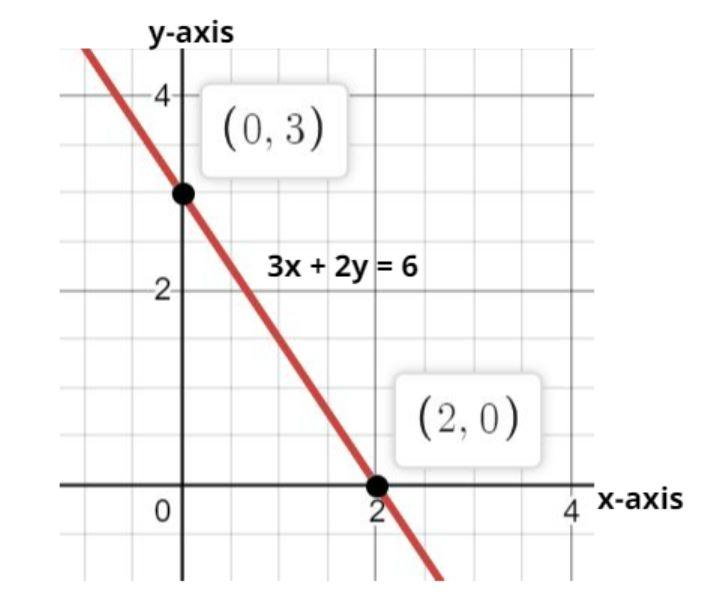Courses
Courses for Kids
Free study material
Free LIVE classes
MoreLIVE
Join Vedantu’s FREE Mastercalss

# Find out the intersection points of the line $3x + 2y = 6$ with x and y axis respectively.$(a){\text{ }}\left( { - 2,0} \right){\text{ and }}\left( {0,3} \right) \\ (b){\text{ }}\left( {2,0} \right){\text{ and }}\left( {0, - 3} \right) \\ (c){\text{ }}\left( {0,2} \right){\text{ and }}\left( {3,0} \right) \\ (d){\text{ }}\left( {2,0} \right){\text{ and }}\left( {0,3} \right) \\$Verified
361.5k+ views
Hint – In this question we have to find the point of intersection of the given line with x axis and y axis. A line cuts x axis at any point (x, 0) as the y-coordinate on x-axis is 0 similarly a line cuts the y-axis at the point (0, y) as the x-coordinate on y-axis is zero. So use this concept to get the point of intersection.Given equation of the line is
$3x + 2y = 6$
Now we have to find out the intersection points of this line with the axis.
Case 1 – Intersection point with x-axis.
As we know on x-axis the value of y is zero.
So, substitute y = 0 in the given equation we have,
$\Rightarrow 3x + 2 \times 0 = 6$
Now simplify this equation we have,
$\Rightarrow 3x = 6 \\ \Rightarrow x = \dfrac{6}{3} = 2 \\$
So, the intersection point with the x-axis is (2, 0) as shown in figure.
Case 2 – Intersection point with y-axis.
As we know on y-axis the value of x is zero.
So, substitute x = 0 in the given equation we have,
$\Rightarrow 3 \times 0 + 2y = 6$
Now simplify this equation we have,
$\Rightarrow 2y = 6 \\ \Rightarrow y = \dfrac{6}{2} = 3 \\$
So, the intersection point with the y-axis is (0, 3) as shown in figure.
So intersection points of the line with the axis are (2, 0) and (0, 3) as shown in figure.
Hence option (d) is correct.

Note –Whenever we face such types of questions the key concept involved is to have a diagrammatic representation of the line given in question, then using the concept that at the point of intersection with any axis or even with any other line the points must satisfy the equation of line. This will help you get on the right track to reach the answer.
Last updated date: 27th Sep 2023
Total views: 361.5k
Views today: 10.61k